Arduino

# How to Automate Your Curtains With ArduinoOctober 09, 2016 by Shizen X
ShareUse an Arduino to make a sound-activated or push-button automated system for your blinds.

## Others

 1 Arduino (I am using Arduino Nano to save space; in the picture, it’s attached to a shield for easy testing) 1 Adafruit sound sensor (optional) 1 Stepper motor + Darlington array driver 3 Buttons 1 LED for indication 0 Jumper wires 1 Breadboard

This Arduino Curtain Automation project will let you automate your curtain blinds using just an Arduino and a stepper motor. I just moved to America pursuing my higher studies. Because my dorm is very dark, I wanted to brighten up my room when I wanted to. Since I would be there for only for a month, I couldn't make anything too complicated. So I decided to make myself this DIY Arduino Curtain Automation system. Using regular curtain blinds, I could control the amount of light in the room and suit it according to my needs.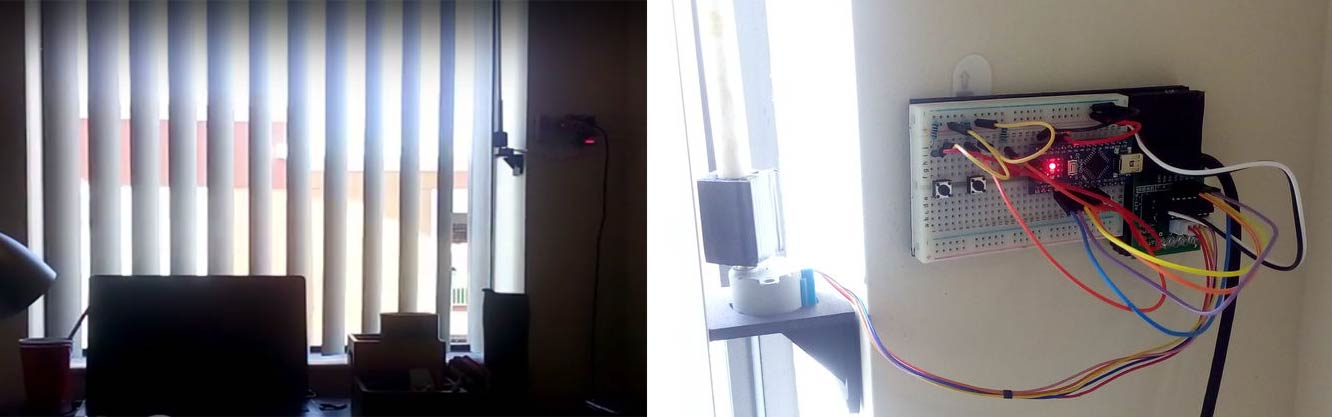The design for this Arduino Curtain Automation system is quite simple and there are two ways to activate the curtains:

Using a sound sensor (mic) to control it using my claps (Step 2).

Using buttons to move the shades of the curtain (Step 3).

So let’s get started with our DIY Arduino Curtain Automation system, follow the simple instructions to replicate this project.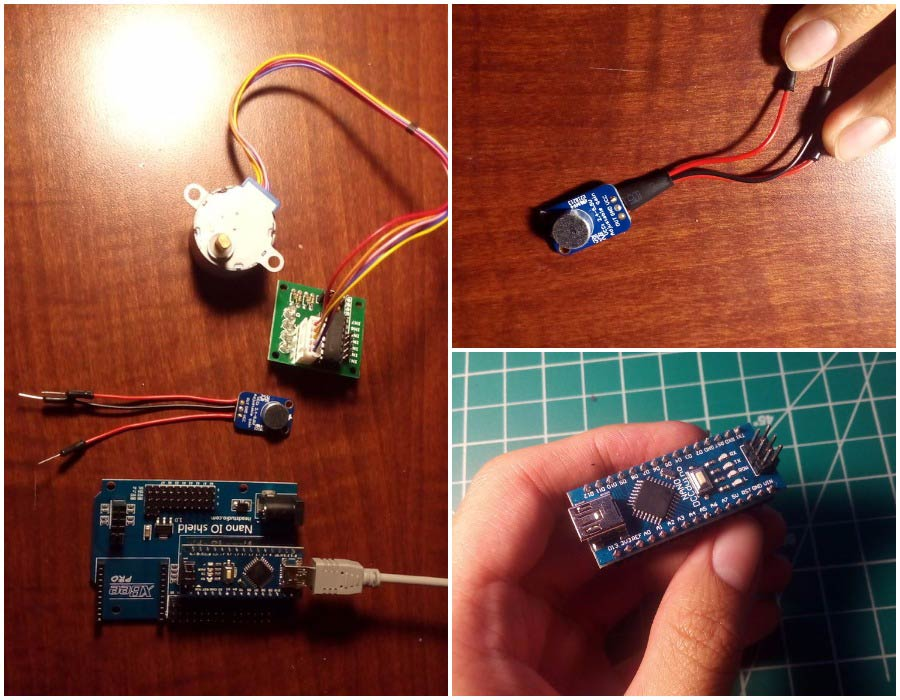How Does the Arduino Curtain Automation Work?The working of this DIY Arduino Curtain Automation system is very simple. The Arduino takes input from either the sound sensor (mic) or buttons. It then correspondingly controls the stepper motor through the Darlington array driver for the motor. The stepper motor is attached to the control stick of the curtain blinds and thus on rotation, opens/closes the blinds.

### Interfacing the Stepper Motor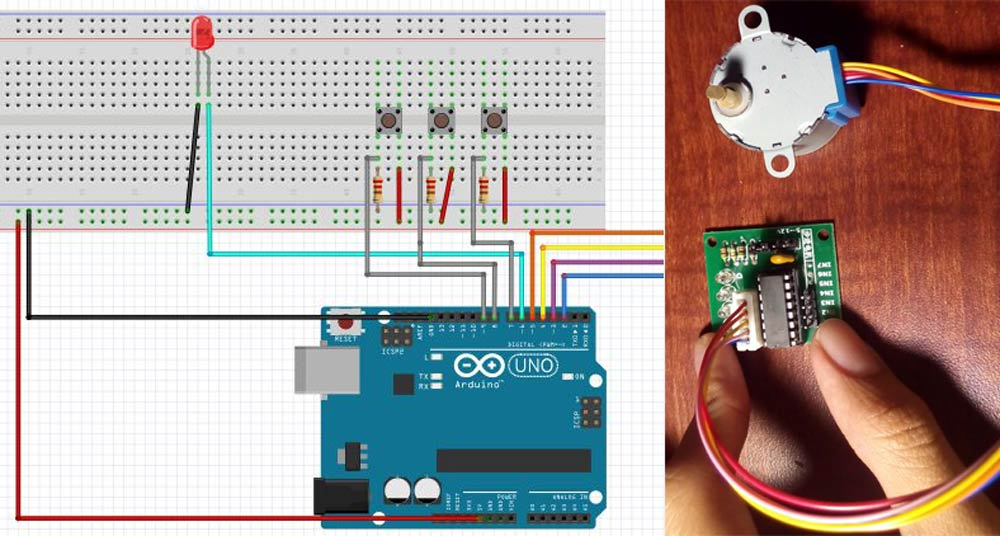The first step I did was testing the design for the Arduino curtain automation system. The motor is connected to the 4 wires of the driver as shown in the diagram. Depending on the direction of rotation for your curtain, you need to plug the driver accordingly.

Basically, the first button from the right will activate the motor to spin a particular degree (you can change in the code). Press the button 4 times and it will return to the original place since it will spin around 90 degrees during each press. The middle button will lock the circuit so that the first button cannot activate the motor. The LED will turn ON when the motor is locked. The final button will return the motor to the original place no matter where is it, at the time pressed. It's still a simple program, hope you guys understand. You can find the code below.

``````int pin={2,3,4,5,6,7,8,9};
int steps[] =
{
{HIGH,HIGH,LOW,LOW},
{HIGH,LOW,LOW,HIGH},
{LOW,LOW,HIGH,HIGH},
{LOW,HIGH,HIGH,LOW},
} ;

int numofroun=1; //Change accordingly to your needs

int current=1;
int type=3;
int place=0;

int lastLockState = LOW;
long lastLockTime = 0;
int LockState;
bool lock=true;

int lastPauseState = LOW;
long lastPauseTime = 0;
int PauseState;
bool Pauseled=false;
bool pause=false;

int lastReturnState = LOW;
long lastReturnTime = 0;
int ReturnState;

void setup() {
for (int num=0; num<5; num++) pinMode(pin[num],OUTPUT);
for (int num=5; num<8; num++) pinMode(pin[num],INPUT);
}

void reset(){
for(int num=0;num<4;num++) digitalWrite(pin[num],LOW);
}

void stepper()
{
for (int num=0; num<4;num++) { digitalWrite(pin[num],steps[abs(type-current)][num]);} if(type==0) {++place;} if(type==3) {--place;} delay(2); } void button1() { Lockreading = digitalRead(pin); if (Lockreading != lastLockState) { lastLockTime = millis(); } if ((millis() - lastLockTime) > 50)
{
if (LockState == HIGH) {
lock=false;
if ((place!=1536*numofroun)&&(place!=1024*numofroun)&&(place!=512*numofroun)) {type=abs(type-3);}
}
}
}
}

void button2()
{
{
lastPauseTime = millis();
}
if ((millis() - lastPauseTime) > 50)
{
if (PauseState == HIGH) {
Pauseled=!Pauseled;
pause=!pause;
if (Pauseled) {digitalWrite(pin,HIGH);}
if (!Pauseled) {digitalWrite(pin,LOW);}
}
}
}
}

void button3()
{
{
lastReturnTime = millis();
}
if ((millis() - lastReturnTime) > 50)
{
if (ReturnState == HIGH) {
type=3;
while (place>0)
{
for (int num=0; num<4;num++) {
digitalWrite(pin[num],steps[3-current][num]);}
--place;
if (current==3) {current=0;}
else ++current;
delay(2);
}
reset();
}
}
}
}

void loop() {
if (lock==true) {button2();button3();}
if (!pause)
{
if (lock==true) {button1();}
if (lock==false) {stepper();}
if ((place==2048)or(place==0)or(((place==1536*numofroun)or(place==1024*numofroun)or(place==512*numofroun))&&(type==3)))
{lock=true;reset();}
if (current==3) {current=0;}
else ++current;
}
}``````

### Setting Up the Arduino Curtain Automation System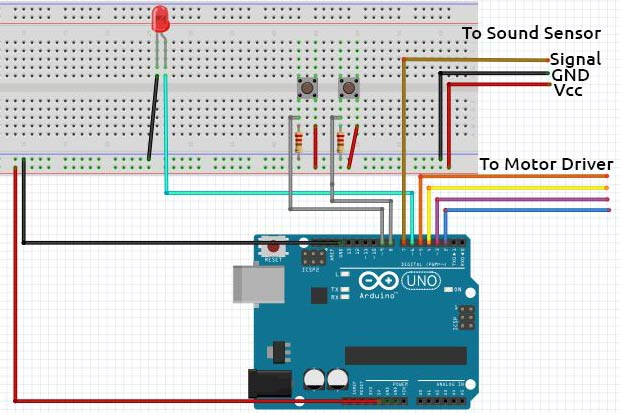After testing the stepper motor, you can use the connection diagram above to make the final prototype. After I finished making mine, I simply changed the input (button) into a sound sensor. You can find the code below.

``````int pin={2,3,4,5,6,7,8,9};
int steps[] =
{
{HIGH,HIGH,LOW,LOW},
{HIGH,LOW,LOW,HIGH},
{LOW,LOW,HIGH,HIGH},
{LOW,HIGH,HIGH,LOW},
} ;

float numofroun=4.5; //Change accordingly to your needs

int current=1;
int type=3;
int place=0;

int claps = 0;
long detectionSpanInitial = 0;
long detectionSpan = 0;
long spancondition;
bool spanconditioncheck=false;

bool lock=true;

int lastPauseState = LOW;
long lastPauseTime = 0;
int PauseState;
bool Pauseled=false;
bool pause=false;

int lastReturnState = LOW;
long lastReturnTime = 0;
int ReturnState;

void setup() {
for (int num=0; num<5; num++) pinMode(pin[num],OUTPUT);
for (int num=5; num<8; num++) pinMode(pin[num],INPUT);
}

void reset(){
for(int num=0;num<4;num++) digitalWrite(pin[num],LOW);
}

void stepper()
{
for (int num=0; num<4;num++) { digitalWrite(pin[num],steps[abs(type-current)][num]);} if(type==0) {++place;} if(type==3) {--place;} delay(2); } void sound() { int sensorState = digitalRead(pin); if (sensorState == 0){ if (claps == 0){ detectionSpanInitial = detectionSpan = millis(); claps++; } else if (claps > 0 && millis()-detectionSpan >= 50){
detectionSpan = millis();
claps++;
}
}
if (millis()-detectionSpanInitial >= 400)
{
if (claps == 2)
{
lock=false;
if ((place!=1024*numofroun)&&(place!=512*numofroun)) {type=abs(type-3);}
spancondition=millis();
}
claps = 0;
}
}

void button1()
{
{
lastPauseTime = millis();
}
if ((millis() - lastPauseTime) > 50)
{
if (PauseState == HIGH) {
Pauseled=!Pauseled;
pause=!pause;
spancondition=millis();
if (Pauseled) {digitalWrite(pin,HIGH);}
if (!Pauseled) {digitalWrite(pin,LOW);}
}
}
}
}

void button2()
{
{
lastReturnTime = millis();
}
if ((millis() - lastReturnTime) > 50)
{
if (ReturnState == HIGH) {
type=3;
while (place>0)
{
for (int num=0; num<4;num++) { digitalWrite(pin[num],steps[3-current][num]);} --place; if (current==3) {current=0;} else ++current; delay(2); } reset(); spancondition=millis(); } } } lastReturnState = Returnreading; } void loop() { if (lock==true) {button1();button2();} if (!pause) { if ((lock==true)&&(millis()-spancondition>1000)) {sound();}
if (lock==false) {stepper();spanconditioncheck=false; }
if ((place==2048*numofroun)or(place==0)or(((place==1024*numofroun)or(place==512*numofroun))&&(type==3))){
lock=true;
reset();
if (!spanconditioncheck){
spancondition=millis();
spanconditioncheck=true;
}
}
if (current==3) {current=0;}
else ++current;
}
}``````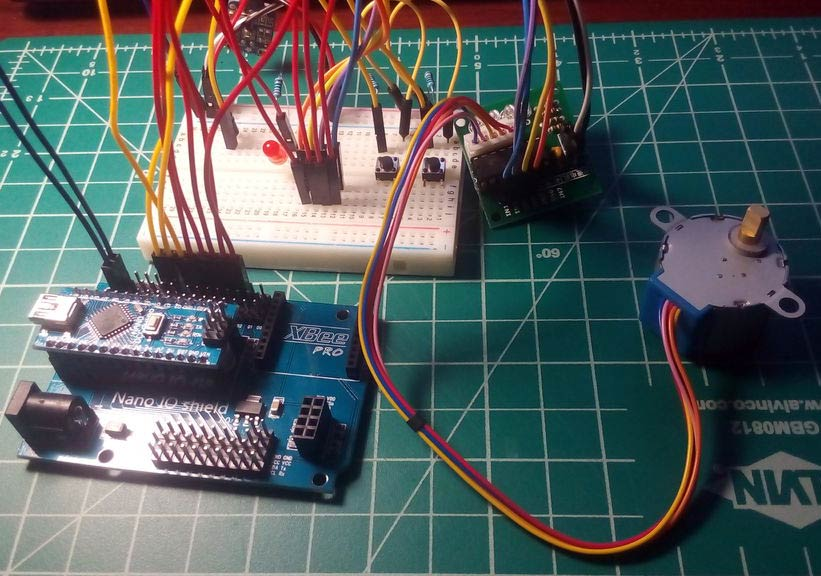I used foam board to make the holder for the motor (1, 2) and curtain handle (3) on my Arduino curtain automation system. Because my dorm doesn't allow modding the wall, I later used double sided tape to stick it in place (1).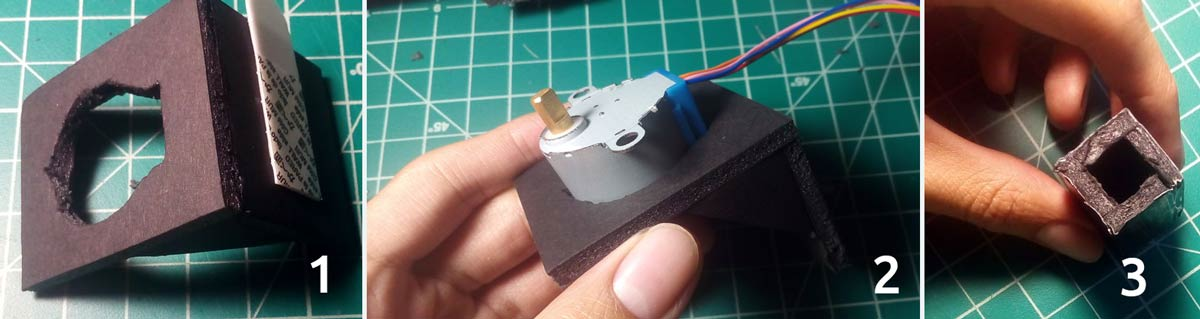The pause button is there so that if your room gets too loud, you can lock it so that the blinds won't go crazy. Note that you might have to adjust the potentiometer on the sound sensor to adjust the sensitivity.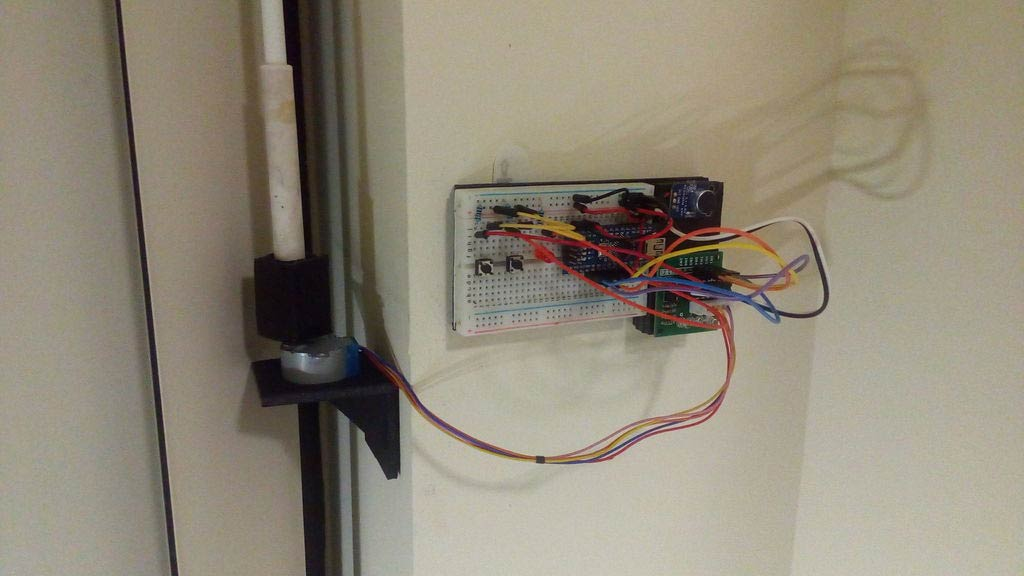Check out the demo video of the clap controlled DIY Arduino Curtain Automation system here:

### Alternate Version Using Buttons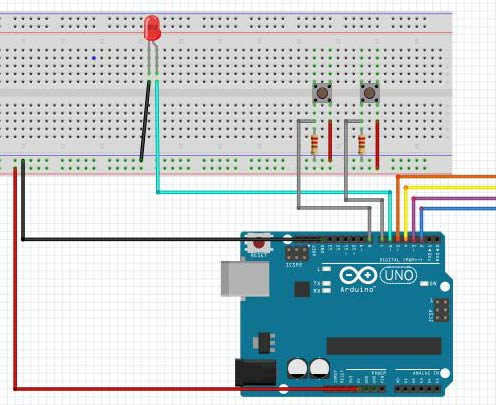If you don't like sound control, you can simply use a button. In this Arduino curtain automation design, there are only two buttons: activate and return (since the pause isn't needed). The activate is the same as before; the reset button will return the curtain back to the original place while activating the LED. You can find the code for this version here.

``````int pin={2,3,4,5,6,7,8};
int steps[] =
{
{HIGH,HIGH,LOW,LOW},
{HIGH,LOW,LOW,HIGH},
{LOW,LOW,HIGH,HIGH},
{LOW,HIGH,HIGH,LOW},
} ;
int current=1;
int type=3;
int place=0;

int lastLockState = LOW;
long lastLockTime = 0;
int LockState;
bool lock=true;

int lastReturnState = LOW;
long lastReturnTime = 0;
int ReturnState;

void setup() {
for (int num=0; num<5; num++) pinMode(pin[num],OUTPUT);
for (int num=5; num<7; num++) pinMode(pin[num],INPUT);
}

void reset(){
for(int num=0;num<4;num++) digitalWrite(pin[num],LOW);
}

void stepper()
{
for (int num=0; num<4;num++) { digitalWrite(pin[num],steps[abs(type-current)][num]);} if(type==0) {++place;} if(type==3) {--place;} delay(2); } void button1() { Lockreading = digitalRead(pin); if (Lockreading != lastLockState) { lastLockTime = millis(); } if ((millis() - lastLockTime) > 50)
{
if (LockState == HIGH) {
lock=false;
if ((place!=1024*4)&&(place!=512*4)) {type=abs(type-3);}
}
}
}
}

void button2()
{
{
lastReturnTime = millis();
}
if ((millis() - lastReturnTime) > 50)
{
if (ReturnState == HIGH) {
type=3;
digitalWrite(pin,HIGH);
while (place>0)
{
for (int num=0; num<4;num++) {
digitalWrite(pin[num],steps[3-current][num]);}
--place;
if (current==3) {current=0;}
else ++current;
delay(2);
}
digitalWrite(pin,LOW);
reset();
}
}
}
}

void loop() {
if (lock==true) {button1();button2();}
if (lock==false) {stepper();}
if ((place==2048*4)or(place==0)or(((place==1024*4)or(place==512*4))and(type==3))){lock=true;reset();}
if (current==3) {current=0;}
else ++current;
}``````

Check out the demo video of this version of my DIY Arduino Curtain Automation system:

I hope that you enjoyed my Android Curtain Automation project. I hope to keep posting more of my projects even though I get quite busy with college. Have fun automating your curtains!